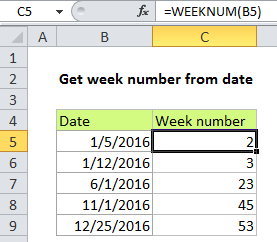# Get week number from date in Excel

If you need to get the week number from the day from a date, you can use the WEEKNUM function. The date must be in a form that Excel recognizes as a valid date.

Note: The WEEKNUM function takes two arguments, a date, and, optionally, an argument called return_type, which controls the scheme used to calculate the week number.## Explanation

In the example, the formula is:

`=WEEKNUM(B5)`

B4 contains a date value for January 5, 2016. The DAY function returns the number 2 representing the calculated week number.

Note that by default, the WEEKNUM function uses a scheme where week 1 begins on January 1, and week 2 begins on the next Sunday (when the return_type argument is omitted, or supplied as 1).

Also See:   Get day from date in Excel

With a return_type of 2, week 1 begins on January 1, and week 2 begins on the next Monday.

### ISO week number

With ISO week numbers, week 1 starts on the Monday of the first week in a year with a Thursday. This means that the first day of the year for ISO weeks is always a Monday in the period between Jan 29 and Jan 4.

Also See:   Sum by week number in Excel

Starting with Excel 2010 for Windows and Excel 2011 for Mac, you can generate an ISO week number using 21 as the return_type:

`=WEEKNUM(date,21)`